• 图像的二维傅里叶变换公式始终没成功推出来，执着地僵持了很久，于是成此文。 二维傅里叶变换的四对公式不清楚可以移步这篇博客的第4种case（因为脉冲阵列就是2维离散周期信号）。 首先应当明确数字图像（M行*N列...

最近复习图像和视频处理课，好多基础推导都不能得心应手，复习进度相当缓慢，过程异常痛苦。图像的二维傅里叶变换的公式始终没成功推出来，执着地僵持了很久，于是成此文。

数字图像（M行*N列）是二维离散信号，可以认为是从一维离散时间信号（也就是 序列）的推广。
(一)二维脉冲阵列信号的FT
考虑二维脉冲阵列信号：
$p(x,y)=\sum_{k=0}^{M-1}\sum_{l=0}^{N-1}\delta(x-k\Delta x, y-l\Delta y)\tag1$
k,l只是序号，实际上$x$只能取离散值$k\Delta x$, $y$只能取$l\Delta y$.
$\Delta x,\Delta y$分别是图像在空域x和y方向的采样间隔。而$\frac{1}{\Delta x},\frac{1}{\Delta y}$是频域u和v方向的采样率.
显然，
$p(x,y)=\delta(x,y)+\delta(x,y-\Delta y)+\cdots+\delta(x,y-(N-1)\Delta y)$
$+\delta(x-\Delta x,y)+\delta (x-\Delta x,y-\Delta y)+\cdots+\delta(x-\Delta x,y-(N-1)\Delta y)+$
$\cdots$
$+\delta(x-(M-1)\Delta x,y-(N-1)\Delta y)\tag2$
所以它是M*N的阵列，每个点的值都是1 .
对（1）做二维FT：（关于二维傅里叶变换公式，移步这里.）
$P(u,v)=\frac{1}{\Delta x}\frac{1}{\Delta y}\sum_{k=0}^{M-1}\sum_{l=0}^{N-1}p(x,y)e^{-j2\pi(uk\Delta x+vl\Delta y)}=\tag3$
直接把（1）进来：
$\frac{1}{\Delta x}\frac{1}{\Delta y}\sum_{k=0}^{M-1}\sum_{l=0}^{N-1}\sum_{k=0}^{M-1}\sum_{l=0}^{N-1}\delta(x-k\Delta x, y-l\Delta y)e^{-j2\pi(uk\Delta x+vl\Delta y)}=\tag4$
由冲激函数的抽样性，只有当$x=k\Delta x,y=l\Delta y$时冲激函数取值为1，则：
$\frac{1}{\Delta x}\frac{1}{\Delta y}\sum_{k=0}^{M-1}\sum_{l=0}^{N-1}e^{-j2\pi(xu+yv)}=\tag5$
由于$x=k\Delta x,y=l\Delta y$，所以：
$\frac{1}{\Delta x}\frac{1}{\Delta y}\sum_{k=0}^{M-1}\sum_{l=0}^{N-1}e^{-j2\pi(k\Delta xu+l\Delta yv)}=\tag6$
由于指数的括号里面的值一定是整数 ，所以$\sum_{k=0}^{M-1}\sum_{l=0}^{N-1}e^{-j2\pi(k\Delta xu+l\Delta yv)}$就是M*N个1，于是可以写成：
$\frac{1}{\Delta x}\frac{1}{\Delta y}\sum_{k=0}^{M-1}\sum_{l=0}^{N-1}\delta(u-\frac{k}{\Delta x},v-\frac{l}{\Delta y})\tag7$
其实（6）—(7) 的过程我不是特别明白，没完全想清楚，如有读者清楚欢迎在评论区说明，谢谢啦。
（二）图像的采样和重建
对图像采样就是原始信号$f(x,y)$与采样脉冲序列$p(x,y)$相乘。
$f_s(x,y)=f(x,y)p(x,y)$
那么在频域就有:
$F_s(u,v)=\frac{1}{2\pi}F(u,v)*P(u,v)=$
$\frac{1}{2\pi}F(u,v)*[\frac{1}{\Delta x}\frac{1}{\Delta y}\sum_{k=0}^{M-1}\sum_{l=0}^{N-1}\delta(u-\frac{k}{\Delta x},v-\frac{l}{\Delta y})]=$
$\frac{1}{2\pi}\frac{1}{\Delta x}\frac{1}{\Delta y}\sum_{k=0}^{M-1}\sum_{l=0}^{N-1}F(u-\frac{k}{\Delta x},v-\frac{l}{\Delta y})$
根据Nyquist定理，只要有
$\frac{1}{\Delta x}\geq2U_{max},\frac{1}{\Delta y}\geq2V_{max}$
原始的模拟信号就可以从采样得到的数字图像中完美重建。
而在实际中，重构是利用低通滤波器实现的。如下图，原始模拟信号经过采样，频谱延拓并周期性重复，用LowPass filter滤出一个完整频谱即可。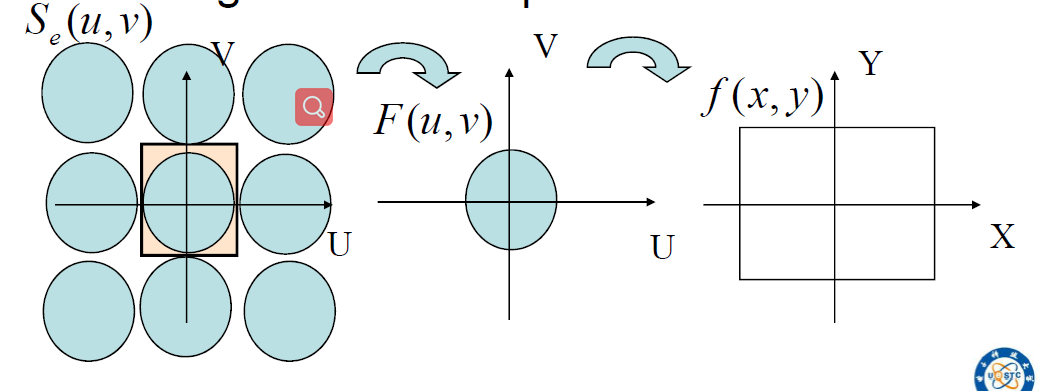展开全文图像采样和量化
• 【图像处理】使用一维DFT叠加实现对图片的二维傅里叶变换 MATLAB 一维DFT 将DFT转化为矩阵运算 参考文档 Gamma公式展示 Γ(n)=(n−1)!∀n∈N\Gamma(n) = (n-1)!\quad\forall n\in\mathbb NΓ(n)=(n−1)!∀n∈N 是...
使用两次一维DFT的叠加方式实现二维的DFT运算可以有效的减少计算成本。
一维DFT公式
$X[k]=\sum_{n=0}^{N-1}x[n]e^{-j\frac{2\pi}{N}kn}$
将DFT转化为矩阵运算
在MATLAB中，使用矩阵运算比循环更加有效率。下面展示对每一行的处理：（以256x256大小图片举例）
对第i行进行DFT处理，则第i行第一个元素的卷积为：
$x[i,1]e^{-j\frac{2\pi}{N}0*0}+x[i,1]e^{-j\frac{2\pi}{N}0*1}+...+x[i,1]e^{-j\frac{2\pi}{N}0*255}$
所以将每行的每个元素都进行此运算之后得到：
$X[i，1]=x[i,1]e^{-j\frac{2\pi}{N}0*0}+x[i,1]e^{-j\frac{2\pi}{N}0*1}+...+x[i,1]e^{-j\frac{2\pi}{N}0*255}\\ X[i，2]=x[i,2]e^{-j\frac{2\pi}{N}1*0}+x[i,2]e^{-j\frac{2\pi}{N}1*1}+...+x[i,2]e^{-j\frac{2\pi}{N}1*2}\\ ...\\...\\ X[i，256]=x[i,256]e^{-j\frac{2\pi}{N}255*0}+x[i,256]e^{-j\frac{2\pi}{N}255*1}+...+x[i,256]e^{-j\frac{2\pi}{N}255*255}$
所以对于图像每一行组成的行向量，指数部分就是：
第1个元素：$0*0+0*1+...+0*255$
第256个元素：$255*0+255*1+...+255*255$
这种加法刚好就构成了一个向量和一个矩阵的乘法过程。
我们构建一个由0到255的列向量和0到255的行向量相乘得到的255x255大小的矩阵。姑且叫做EMatrix。
$e^{-j\frac{2\pi}{N}}\begin{bmatrix} 0\\ ...\\ 255 \end{bmatrix} *\begin{bmatrix} 0 & ... & 255 \end{bmatrix}=e^{-j\frac{2\pi}{N}}\begin{bmatrix} 0*0& 0*1 & ... &0*255\\ ...& ...& ...& ...\\ ...& ...& ...& ...\\ 255*0& ...& ...&255*255 \end{bmatrix}$
所以对于图片的每一行，第一个元素会对应乘上矩阵的第一行的每个元素并相加，得到对应的第一元素的X[k]，将每一行都乘以这个矩阵就可以得到对图片进行行处理的结果。
$X[i,:]=x[i,:]*EMatrix=\begin{bmatrix} x[i,1] & ... & ... & x[i,256] \end{bmatrix}* e^{-j\frac{2\pi}{N}}\begin{bmatrix} 0*0& 0*1 & ... &0*255\\ ...& ...& ...& ...\\ ...& ...& ...& ...\\ 255*0& ...& ...&255*255 \end{bmatrix}$
下一步就是对图片的每一列进行相同的步骤，不过注意列向量拿出来之后需要转置再乘EMatrix再转置得到对应列的结果。
MATLAB代码
%% Q2 1D-DFT
raw_image=imread('hh.jpg'); % read image
image=im2double(rgb2gray(raw_image));
[M,N]=size(image);
one_d1=zeros(M,N); % x-dimention
one_d2=zeros(M,N);% y-dimention
k=0:1:255;
n=0:1:255;
kn=k'*n; % get exp kernel
E=exp(-1i*2*pi/256).^(kn);
tic % start counting
for a=1:M % for every row
one_d1(a,:)=image(a,:)*E;
end
for b=1:N % for every column
one_d2(:,b)=(one_d1(:,b)'*E)';
end
toc % stop counting
subplot(1,2,1),imshow(raw_image),title('raw');
subplot(1,2,2),imshow(one_d2),title('1D-DFT');

用我最喜欢的憨憨龟来测试一下用时0.014302秒，相比于直接用公式计算2D的DFT要快非常多。
提到傅里叶变换就不得不提到快速傅里叶变换FFT，在我下一篇文章里有介绍。


展开全文matlab 线性代数 信号处理
• ## 二维DFT

千次阅读 2014-11-17 15:43:04
学习DIP第4天 傅里叶变换数学原理会在后续完整介绍，目前只实现代码，观察下结果，公式在上一篇博客中已经描述  内容迁移至 http://www.face2ai.com/DIP-2-2-二维...http://www.tony4ai.com/DIP-2-2-二维DFT/...
学习DIP第4天傅里叶变换数学原理会在后续完整介绍，目前只实现代码，观察下结果，公式在上一篇博客中已经描述

内容迁移至

http://www.face2ai.com/DIP-2-2-二维DFT/

http://www.tony4ai.com/DIP-2-2-二维DFT/
展开全文数字图像处理 c语言
• 将Gunther提出的关于同时计算一个N点实序列的DFT和另一个N点实序列的DFT的逆离散傅里叶变换（IDFT）的4个新的直接公式中的第1和第4个公式，以及他提出的关于同时计算2个N点实序列的DFT的新公式推广到了二维的情形，...
• 图像处理 二维离散傅里叶变换DFT matlab代码图像处理领域离散傅里叶变换的作用二维离散傅里叶变换二维离散傅里叶变换公式二维的离散傅里叶变换进行转化将系数转化为矩阵形式注意，从矩阵的乘积i形式可以看出，原来...
图像处理 二维离散傅里叶变换DFT matlab代码图像处理领域离散傅里叶变换的作用二维离散傅里叶变换二维离散傅里叶变换公式将二维的离散傅里叶变换进行转化将系数转化为矩阵形式注意，从矩阵的乘积i形式可以看出，原来是N个值，输出后依然是N个值，而且要注意，输出的N个值的每一个值和输入得每一个值都有关系。换句话说，输出的F(0),F(1),F(2)....F(N)，之中的每一个值，比如F(1)，都需要，输入的所有值做贡献。f(x)的全部值对离散的傅氏变换四项中的每一项都产生影响。反之亦然。matlab代码
图像处理领域离散傅里叶变换的作用
将图像由时空域，转换为频域
二维离散傅里叶变换
二维离散傅里叶变换公式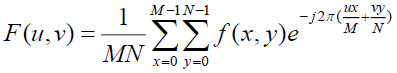将二维的离散傅里叶变换进行转化
将不好处理的二维离散傅里叶变换转化为进行两次一维处理
先处理行，再处理列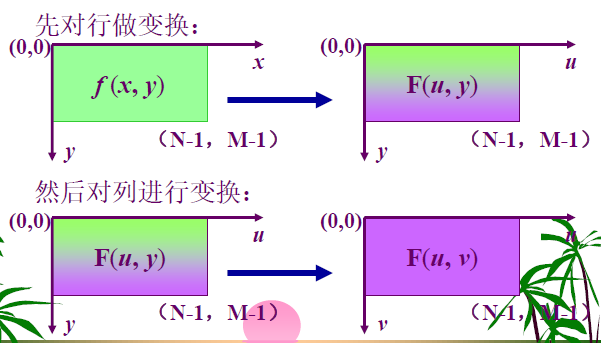公式如下：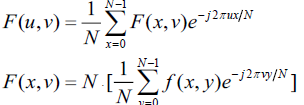将系数转化为矩阵形式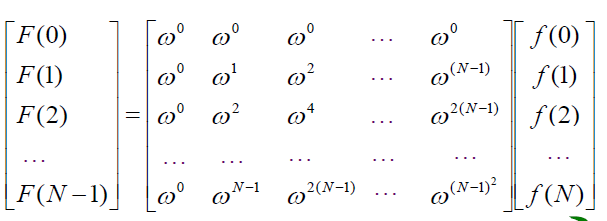注意，从矩阵的乘积i形式可以看出，原来是N个值，输出后依然是N个值，而且要注意，输出的N个值的每一个值和输入得每一个值都有关系。换句话说，输出的F(0),F(1),F(2)…F(N)，之中的每一个值，比如F(1)，都需要，输入的所有值做贡献。
f(x)的全部值对离散的傅氏变换四项中的每一项都产生影响。反之亦然。
所以按照刚才将维的思路，进行矩阵的操作。
权值矩阵A，原函数矩阵FF
采用88的DFT操作，对每一个88图像块，进行一次操作
matlab代码
N=8;
A=zeros(N);
for k=0:N-1
for m=0:N-1
A(k+1,m+1)=(1/N)*exp(-2*pi*i*(k*m/N));%8*8的 w 的矩阵
end
end

[Hh,Hw]=size(WYY);
X=ceil(Hw/8)*8;
Y=ceil(Hw/8)*8;
hall_new=zeros(X,Y);
hall_new(1:Hh,1:Hw)=WYY;%把Y分量赋值进去
%hall_new(Hh+1:X,1:Hw)=repmat(WYY(Hh,1:Hw),X-Hh,1);
%hall_new(1:Hh,Hw+1:Y)=repmat(WYY(1:Hh,Hw),1,Y-Hw);
m=X/8;%行块数
n=Y/8;%列块数
for i1=1:m
for i2=1:n
FF=double(hall_new((i1-1)*8+1:i1*8,(i2-1)*8+1:i2*8));%按顺序取8*8的每一块
BB((i1-1)*8+1:i1*8,(i2-1)*8+1:i2*8)=N*A*FF*A';%DFT变换，变成两次一维度处理，另一个函数，先处理一行，一行的8个值都会产生影响，以行和列为整体看
KK((i1-1)*8+1:i1*8,(i2-1)*8+1:i2*8)=A'*BB((i1-1)*8+1:i1*8,(i2-1)*8+1:i2*8)*A;%DFT反变换
end
end

未完待续


展开全文图像处理代码 matlab
• ## DCT变换和DFT变换

千次阅读 2018-12-16 16:45:44
这里我只谈论二维DFT变换，有关一维的DFT，请查看一下博客，里面有详细介绍： https://blog.csdn.net/IMWTJ123/article/details/79831242 根据上面的公式，我们可以得到对应的离散傅里叶变换呃代码，下面是...IDFT JPEG
• 二维DFT公式： 。 可以看作M行N列的二维数组，先对每行做一维FFT，将结果作为一个新的二维数组。再对新的二维数组每列做一维FFT。而在处理二维IFFT的时候，跟二维FFT差不多，只要在公共方法中控制正负值，来区分...FFT 快速傅里叶
• 二维DFT公式： 。 可以看作M行N列的二维数组，先对每行做一维DFT，将结果作为一个新的二维数组。再对新的二维数组每列做一维DFT。 而在处理二维IDFT的时候，跟二维DFT差不多，只要在公共方法中控制正负值，来...
• 1.离散FFT简单介绍  FFT是一种DFT的高效算法，称为快速傅立叶变换（fast Fourier transform）。其原理比较复杂，我们可以不...二维FFT变换公式如下： 2.复数工具类  FFT和二维FFT都需要复数的加减乘除，在这里给java
• ## 傅里叶变换二维离散傅里叶变换

万次阅读 热门讨论 2019-11-07 15:41:28
DFT:(Discrete Fourier Transform)离散傅里叶变换是傅里叶变换在时域和频域上都呈离散的形式，将信号的时域采样变换为其DTFT的频域采样。在形式上，变换两端（时域和频域上）的序列是有限长的，而实际上这两组序列...
•   前面提到了单变量的离散傅里叶变换，但是对于图像来说，是一个二维的坐标系，因此在对图像进行傅里叶变换时，对其进行的是二维的离散傅里叶变换，与一维DFT类似，给出二维的DFT的公式： 其中，f(x,y)是大小为M*N...
• 例题 计算以下图像的离散傅里叶变换 f= f=\begin{bmatrix}1&4&4&1\\2&4&4&2\\2&...f=⎣⎢⎢⎡​1221​4444​...考虑二维DFT的分解： F(u,v)=∑x=0M−1∑y=0N−1f(x,y)e−
• 二维离散傅里叶变换(DFT) 其中f(x,y)为原图像，F(u,v)为傅里叶变换以后的结果，根据欧拉公式可得，每个F(u,v)值都为复数，由实部和虚部组成 代码示例 void dft(short** in_array, double** re_array.
• 《数字图像处理》第三版 Rafael C. Gonzalez等著 花了1，2个月断续的看书，终于有些进展了，一直到DFT这里，偏理论些。...按照公式F(u,v)，可以看出来每个点的值是一个复数，假设F(u0,v0)=a + b j；书图像处理 傅立叶
• ## 傅里叶变换和正弦函数和欧拉公式

万次阅读 多人点赞 2017-06-27 15:43:51
以及它们在傅里叶变换中的作用，附加：傅里叶变换和卷积公式这是我第二次学习傅里叶变换，其实第一次就已经懂了时域和频域的关系，也知道一维傅里叶变换就是将一个函数转化为很多频率不同的正弦函数的和，二维图片...正弦函数
• 第5章 Core组件进阶 5.5 离散傅里叶变换（Discrete Fourier Transform，DFT） 5.5.1 离散傅里叶变换原理 1.对一张图像使用傅里叶变换就是把它分解成正弦和余弦，将图像...3.二维图像的傅里叶变换数学公式表达：,...OpenCV
• 码字不易，如果对您有所帮助，记着点赞哦！... 对二维图像进行傅里叶变换用如下公式进行： 图像长M，高N。F(u,v)表示频域图像，f(x,y)表示时域图像。u的范围为[0,M-1]，v的范围为[0,N-1] ↑ 对二维图...python源码 数字图像处理
• 1、原理2、实现 ...二维图像的傅立叶变换可以用以下数学公式表达： 式中f(i, j)是图像空间域的值而F是频域的值。傅立叶转换的结果是复数，这也显示出了傅立叶变换是一副实数图像（real image）和虚数图像（..opencv
• 图像的正交变换在数字图像的...二维 DFT 的变换及逆变换公式如下： DFT 尽管解决了频域离散化的问题，但运算量太大。从公式中可以看到，有两个嵌套的求和符号，显然直接计算的复杂度为O(n2)O(n2)。为了加快傅里..
• 图像的正交变换在数字图像的处理与分析中起...二维 DFT 的变换及逆变换公式如下： DFT 尽管解决了频域离散化的问题，但运算量太大。从公式中可以看到，有两个嵌套的求和符号，显然直接计算的复杂度为$$O(n^2)$$。...
• 二维离散傅里叶变换DFT公式如下： 可以看到DFT中包含了复数运算，计算复杂度高。然而实际应用中，信号大多数都是实数域上的，使用DFT会造成很大的冗余。 根据欧拉公式，一个复指数信号可以分为实部和虚部两...DCT变化
• 使用FFTW和简单的朴素算法计算一维和二维离散傅立叶变换DFT），以明确说明FFTW在其输入和输出中包含什么样的对称性和缩放属性。 一维示例 本教程首先计算随机输入数据的一维（1D）DFT。 一维复数到复数 第一个示例...
• DCT变换，离散余弦变换，将时域/空间域转换到频域上。是DFT离散的傅里叶变换去掉虚数项的表示。...二维DCT变换： 矩阵表示和matlab程序 分块DCT变换 https://www.cnblogs.com/wyuzl/p/7880124.html ...
• 1. 计算一维离散傅里叶变换DFT公式如下： 其中，N表示数据长度。由上式可知，DFT的时间复杂度是O(N*N) 2. 一维FFT的时间复杂度为O(N*logN)，其中N表示数据长度 3. 对于一个M*N的二维数据，FFT的时间...
• 通过以上公式处理后可以实现图像的二维傅立叶变换，但时间复杂度非常高，因此本次实验我采用了快速傅立叶变换，把时间复杂度大大降低，公式如下： 快速傅里叶变换（FFT）是计算离散傅里叶变换DFT）的 DFT的定义式...